Courses

# Test: General Properties Of d-Block Elements

## 25 Questions MCQ Test Chemistry for JEE | Test: General Properties Of d-Block Elements

Description
This mock test of Test: General Properties Of d-Block Elements for JEE helps you for every JEE entrance exam. This contains 25 Multiple Choice Questions for JEE Test: General Properties Of d-Block Elements (mcq) to study with solutions a complete question bank. The solved questions answers in this Test: General Properties Of d-Block Elements quiz give you a good mix of easy questions and tough questions. JEE students definitely take this Test: General Properties Of d-Block Elements exercise for a better result in the exam. You can find other Test: General Properties Of d-Block Elements extra questions, long questions & short questions for JEE on EduRev as well by searching above.
QUESTION: 1

### Only One Option Correct Type Direction (Q. Nos. 1-10) This section contains 10 multiple choice questions. Each question has four choices (a), (b), (c) and (d), out of which ONLY ONE is correct. Q.  Atomic number of second transition series lies from

Solution:

Since,1st transition series belongs to 3d orbital and 2nd transition series belongs to 4d-orbital. According to the given option, atomic number 39 to 48 (5s24d1-10) belongs to second transition series.

QUESTION: 2

### Which has lower standard reduction potential (SRP) value?

Solution:

Mn2+/ Mn have - 1.18SRPvalue.

QUESTION: 3

### An element has configuration 4d55s2. The element belongs to

Solution:

Ford-block elements, group number is equal to the number of electrons in (n - 1)d subshell + number of electrons in valence shell (nth shell).

QUESTION: 4

The electronic configuration of palladium is

Solution:

[Kr]4d105s0

QUESTION: 5

Which has lowest and highest first ionisation enthalpy in 3d series?

Solution:

Sc (3d4s2)has 631 kJ mol-1 and Zn (3d10 4s2) has 906 kJ mol-1

QUESTION: 6

The electronic configuration of the element which is just above the element with atomic number 42 in the same group

Solution:

The element lying above Z = 42 has Z value 24, i.e. chromium. Hence, Cr configuration is [Ar]3d54s1

QUESTION: 7

Which one of the following exists in the oxidation state other than +3?

Solution:

The correct answer is option C

Ce exist in the oxidation state other than +3 .

(a) B - Boron has +1 and +3 oxidation state.

(b) Al - Aluminium has +1,+2 and +3 oxidation state.

(c) Ce - Cerium has +3 and +4 oxidation state.

(d) Ga - Gallium has +1 and +3 oxidation state.

Cerium (Ce) have [Xe] 4f²6s² electronic configuration.

QUESTION: 8

Electronic configuration of a transition element X in +3 oxidation state is [Ar] 3d5 and Y in +2 state is [Ar] 3d8. What are the atom ic numbers of the elements?

Solution:

Fe (Z = 26) in + 3 oxidation state show Fe3+ ([Ar] 3d5) configuration. Ni(28) in +2 oxidation state show Ni2+([Ar]3d8) configuration.

QUESTION: 9

The atomic radii of Cu and Ag are 1.17 Å and 1.34 Å. The atomic radius of gold is expected to be

Solution:

Due to lanthanide contraction, Ag and Au have nearly same size.

QUESTION: 10

A red solid is insoluble in water. However, it becomes soluble if some KI is added to water. Heating the red solid in a test tube results in liberation of some violet coloured fumes and droplets of a metal appear on the cooler parts of the test tube. The red solid is

Solution: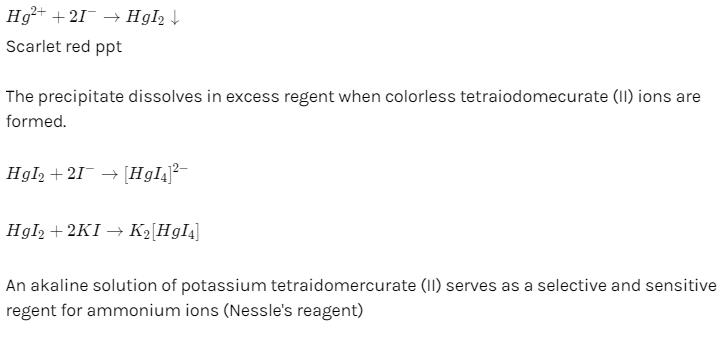*Multiple options can be correct
QUESTION: 11

One or More than One Options Correct Type

Direction (Q. Nos. 11-15) This section contains 5 multiple choice questions. Each question has four choices (a), (b), (c) and (d), out of which ONE or MORE THAN ONE are correct.

Q.

Identify the pairs in which one covalent radii of elements are almost similar.

Solution:

Due to lanthanide contraction.

QUESTION: 12

Which one is of the following is the lightest transition element?

Solution:

The correct answer is Option B.

Sc has the lowest density (3.1 g cm−3). It is the lightest transition element.

*Multiple options can be correct
QUESTION: 13

Which of the following have pseudo noble gas configuration?

Solution:

An element with pseudo noble gas configuration has 18 electrons in the highest energy level instead of 8.
Zn2+(28) = 3s2, 3p6, 3d10
Ag+ (46) = 4s24p64d10

*Multiple options can be correct
QUESTION: 14

Which one of the following is a diamagnetic ion?

Solution:

The correct answer is option A,D.
About two–thirds of all metals crystallize in closest-packed arrays with coordination numbers of 12. Metals that crystallize in an HCP structure include Cd, Co, Li, Mg, Na, and Zn, and metals that crystallize in a CCP structure include Ag, Al, Ca, Cu, Ni, Pb, and Pt.

*Multiple options can be correct
QUESTION: 15

The pair of ions that have same electronic configuration

Solution:

Both Fe2+ and Co3+ have [Ar] 3d6 configuration while V2+ and Cr3+ have [Ar] 3d3.

QUESTION: 16

Comprehension Type

Direction (Q. Nos. 16 and 17) This section contains a paragraph, describing theory, experiments, data, etc. Two questions related to the paragraph have been given. Each question has only one correct answer among the four given options (a), (b), (c) and (d).

Passage

The atomic and ionic radii for transition elements are smaller than their corresponding s-block elements and are greater than their corresponding p-block elements. The atomic and ionic radii transition elements for a given series show decreasing trend for first elements constant in the middle and slight increase towards the end. This is due to the combined effect of increasing effective nuclear charge and increasing screening effect along the period.
The atomic and ionic radii of 4d series are greater than 3d series. However, the elements of 4d series and 5d series have almost similar sizes due to lanthanide contraction. Transition elements have low atomic volumes, high densities, high melting points.

Q.

Which pair of elements has nearly same atomic size?

Solution:

Due to lanthanide contraction.

QUESTION: 17

Passage

The atomic and ionic radii for transition elements are smaller than their corresponding s-block elements and are greater than their corresponding p-block elements. The atomic and ionic radii transition elements for a given series show decreasing trend for first elements constant in the middle and slight increase towards the end. This is due to the combined effect of increasing effective nuclear charge and increasing screening effect along the period.
The atomic and ionic radii of 4d series are greater than 3d series. However, the elements of 4d series and 5d series have almost similar sizes due to lanthanide contraction. Transition elements have low atomic volumes, high densities, high melting points.

Q.

The metal with high melting point in 3d series is

Solution:

The high melting point of Cr metal is attributed to the involvement of greater number of electrons from (n - 1) d in addition to ns electrons in the interatomic metallic bonding.

QUESTION: 18

Matching List Type

Choices for the correct combination of elements from Column I and Column II are given as options (a), (b), (c) and (d), out of which one is correct.

Q.

Match the Column I with Column II and mark the correct option from codes given below.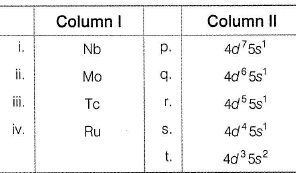Solution:

(i) → (s), (ii) → (r), (iii) → (q), (iv) → (p)

QUESTION: 19

Which one of the following ions exhibits colour in aqueous solution

Solution: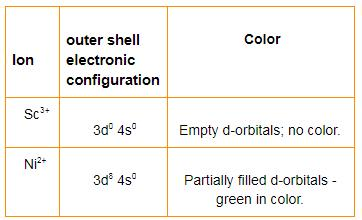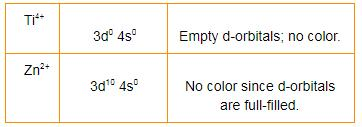*Answer can only contain numeric values
QUESTION: 20

One Integer Value Correct Type

Direction (Q. Nos. 20-23) This section contains 4 questions. When worked out will result in an integer from 0 to 9 (both inclusive).

Q.

The number of elements that do not have completely filled d subshell among the elements Ni, Pd, Pt, Cu, Ag, Au, Zn, Cd and Hg are

Solution:

Ni has 3d84s2 and Pt has 5d96s1.

*Answer can only contain numeric values
QUESTION: 21

Total spin of the electrons in a chromium atom is

Solution:

Chromium has 6 unpaired electrons and hence, its spin value is - 3 or +3. Cr,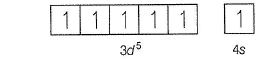There are 6 unpaired electrons in chromium.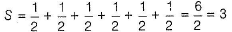*Answer can only contain numeric values
QUESTION: 22

Number of electrons with l = 2 and s = 1/2 in zinc atom are

Solution:

l = 2 means d-subshell. Zinc has 10 electrons ind-subshell in which 5 electrons are + 1/2 spin and 5 electrons are - 1/2.

*Answer can only contain numeric values
QUESTION: 23

Number of elements that generally do not show variable valencies Cu, Zn Au, Cd, Sc, La, Pt, Co.

Solution:

Zn, Cd, Sc and La do not show variable valencies.

QUESTION: 24

During the process of electrolytic refining of copper, some metals present as impurity settle as ‘anode mud’ These are

Solution:

The correct answer is option C
In Electrolytic refining, the impure metal is made to act as an anode. A strip of the same metal in pure form is used as a cathode.
They are put in a suitable electrolytic bath containing a soluble salt of the same metal.
The more basic metal remains in the solution and the less basic ones go to the anode mud.
Copper is refined using an electrolytic method.
Impurities from the blister copper deposit as anode mud which contains antimony, selenium, tellurium, silver, gold, and platinum; recovery of these elements may meet the cost of refining.

QUESTION: 25

Statement Type

Direction (Q. No. 25) This section is based on Statement I and Statement II. Select the correct answer from the codes given below.

Q.

Statement I : The highest oxidation state of osmium is +8.

Statement II : Osmium is a 5-d block element.

Solution:

Osmium has the electronic configuration 5de6s2. As 5d and 6s are close in energy, all the 8 electron can participate in bonding. highest oxidation state of osmium is +8 due to its ability to expand their octet by using its all 8 electrons (2 from 6s and 6 from 5d).